# Poisson window

The coefficients of the Poisson window, also called the exponential window, are given by the following formula

$$a(k)=e^{-\alpha \frac{|k-M|}{M}}$$

where N is the length of the filter, M = (N – 1) / 2, k = 0, 1, …, N – 1, and, typically, 0 ≤ α < 1. α is often set to 2 D / 8.69, were D is the desired decay of the window in decibels over half of the window length.

The multiplication of the Poisson window with the Hann window produces the hybrid Hann-Poisson window.

Consider a finite impulse response (FIR) low pass filter of length N = 201. The following is the Poisson window with α = 0.5.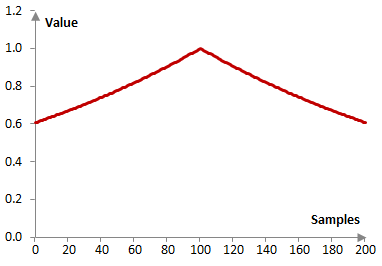Given a sampling frequency of 2000 Hz and a filter cutoff frequency of 40 Hz, the impulse response of the filter with a rectangular window (with no window) and with the Poisson window is as follows.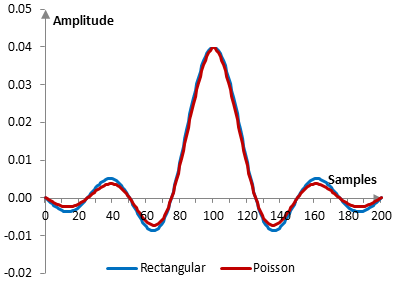The magnitude response of the same filter is shown on the graph below.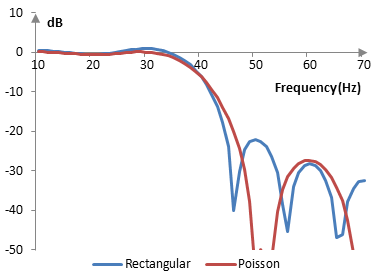As the parameter α becomes larger, the transition band of the filter with the Poisson window becomes wider and the Gibbs phenomenon ripples in the pass band become smaller. At α = 0, the Poisson window becomes the rectangular window.

The following is the Poisson window with N = 201 and at three different values of α (0.2, 0.5, and 0.8).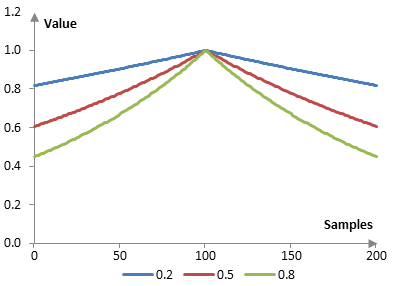The magnitude response of a filter at these values of α, given a sampling frequency of 2000 Hz and a cutoff frequency of 40 Hz, is shown below.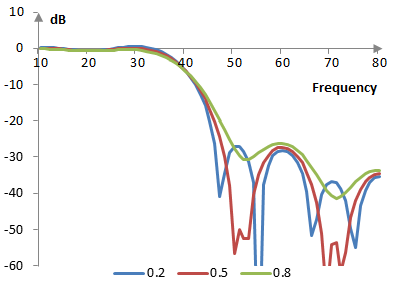## Measures for the Poisson window

The following is a comparison of the discrete Fourier transform of the Poisson window (α = 0.4) and the rectangular window.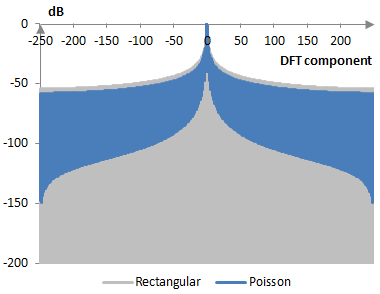The Poisson window measures are as follows.

 α 0.2 0.5 0.8 Coherent gain 0.91 0.79 0.69 Equivalent noise bandwidth 1.00 1.02 1.05 Processing gain -0.01 dB -0.09 dB -0.22 dB Scalloping loss -3.69 dB -3.36 dB -3.05 dB Worst case processing loss -3.70 dB -3.45 dB -3.27 dB Highest sidelobe level -14.7 dB -17.5 dB -19.9 dB Sidelobe falloff -6.1 dB / octave, -20.3 dB / decade -6.3 dB / octave, -20.8 dB / decade -6.4 dB / octave, -21.4 dB / decade Main lobe is -3 dB 0.90 bins 0.94 bins 1.00 bins Main lobe is -6 dB 1.24 bins 1.30 bins 1.36 bins Overlap correlation at 50% overlap 0.497 0.480 0.450 Amplitude flatness at 50% overlap 0.995 0.970 0.925 Overlap correlation at 75% overlap 0.773 0.794 0.799 Amplitude flatness at 75% overlap 0.999 0.992 0.980

Window

### Filtered HTML

• Freelinking helps you easily create HTML links. Links take the form of [[indicator:target|Title]]. By default (no indicator): Click to view a local node.